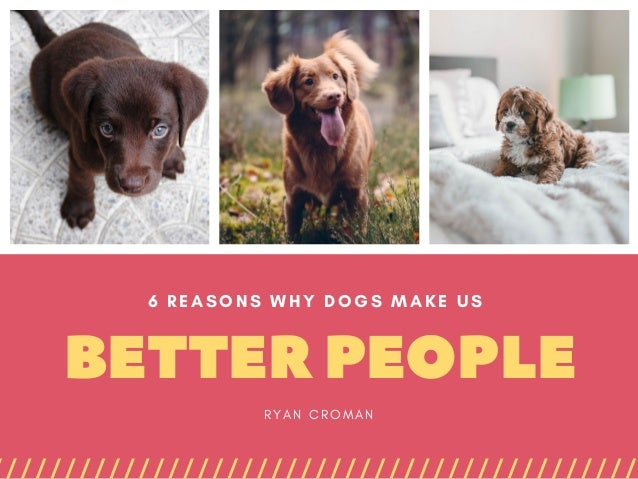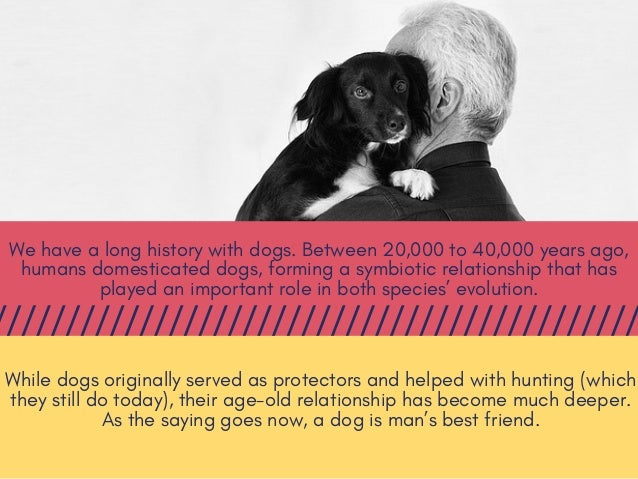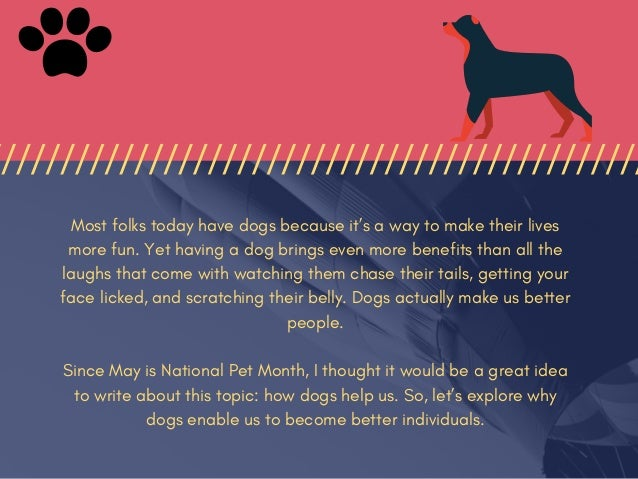Successfully reported this slideshow.Upcoming SlideShare
×

ofUpcoming SlideShare
Next

0 Likes

Share

# Dogs Make Us Better People

We have a long history with dogs. Between 20,000 to 40,000 years ago, humans domesticated dogs, forming a symbiotic relationship that has played an important role in both species’ evolution.

See all

### Related Audiobooks

#### Free with a 30 day trial from Scribd

See all
• Be the first to like this

### Dogs Make Us Better People

1. 1. BETTERPEOPLE 6 REASONS WHY DOGS MAKE US  R Y A N C R O M A N
2. 2. We have a long history with dogs. Between 20,000 to 40,000 years ago, humans domesticated dogs, forming a symbiotic relationship that has played an important role in both species’ evolution. While dogs originally served as protectors and helped with hunting (which they still do today), their age-old relationship has become much deeper. As the saying goes now, a dog is man’s best friend.
3. 3. Most folks today have dogs because it’s a way to make their lives more fun. Yet having a dog brings even more benefits than all the laughs that come with watching them chase their tails, getting your face licked, and scratching their belly. Dogs actually make us better people. Since May is National Pet Month, I thought it would be a great idea to write about this topic: how dogs help us. So, let’s explore why dogs enable us to become better individuals.
4. 4. HAVINGADOGIMPROVES PATIENCE E v e r y p e r s o n w h o h a s a d o g h a s h a d t o d e a l w i t h p o o p o n t h e f l o o r o r s o m e t h i n g g e t t i n g t o r n u p ( n o t m y n e w s o c k s ! ) . W h a t t h i s b u i l d s i n y o u i s p a t i e n c e . W i t h a d o g , y o u l e a r n h o w t o d e a l w i t h u n i d e a l s i t u a t i o n s a n d f i g u r e o u t w a y s t o t r a i n a n d i m p r o v e t h e d o g ’ s b e h a v i o r . N o w o n d e r 5 4 % o f d o g p a r e n t s s a y t h e i r p u p h a s m a d e t h e m m o r e p a t i e n t , a c c o r d i n g t o a B a r k B o x s t u d y .
5. 5. HAVINGA DOGMAKES USHAPPY That same BarkBox study found that 71% of dog parents say their pup has made them happier. There’s a variety of reasons that dogs enhance our happiness. They offer unconditional love and are always there for us. They encourage us to exercise and get outside in nature, keeping us physically healthy and keeping our endorphins and Vitamin D up (which keeps us mentally healthy). Dogs also teach us how to live in the moment, as their excitement over a butterfly in the park or playing fetch encourages us to forget all else and just have a good time. The interesting thing is that Harvard researchers have discovered that happiness is actually contagious. So, the happiness our dogs bring us will actually spread to the friends and family around us. That’s a powerful thing.
6. 6. HAVINGADOG MAKESUSMORE RESPONSIBLE W e a r e c a r i n g f o r a n o t h e r l i v i n g t h i n g . T h i s c o m e s w i t h t h e r e s p o n s i b i l i t y t o e n s u r e i t s p h y s i c a l a n d m e n t a l w e l l b e i n g . I f t h a t m e a n s g e t t i n g u p a t 4 a . m . t o t a k e t h e p u p o u t s i d e t o p e e , y o u g e t u p a n d d o i t . A c c o r d i n g t o t h e B a r k B o x s t u d y , 5 2 % o f d o g p a r e n t s b e l i e v e t h e y ’ v e b e c o m e m o r e r e s p o n s i b l e s i n c e g e t t i n g a d o g . I b e l i e v e I h a v e a s w e l l .
7. 7. HAVINGADOGMAKESUS MOREAFFECTIONATE The BarkBox survey discovered that about 47% of dog parents feel they’ve learned more about affection from their dog. This isn’t surprising, considering that dogs will cuddle with you when you’re feeling down or jump and play with you when you’re feeling good. Being more affectionate can lead to stronger relationships with friends and family. This is because you’ll be more in tune with your emotions and understand more about how to be a caring individual.
8. 8. HAVINGADOGMAKES YOUABETTER COMMUNICATOR M u c h o f c o m m u n i c a t i o n i s n o n v e r b a l , r e s e a r c h h a s s h o w n . A s f a r a s I k n o w , t h e r e a r e n ’ t a n y d o g s t h a t c a n t a l k , t h o u g h t h e y c a n u n d e r s t a n d m a n y w o r d s . W h a t t h i s m e a n s i s t h a t w e m u s t u s e o t h e r w a y s t o c o m m u n i c a t e , s u c h a s t o n e o f v o i c e a n d n o n - v e r b a l s i g n a l s l i k e t o u c h i n g a n d g e s t u r i n g . Having to do this with our dogs encourages us to be more focused on our body language and tone of voice in interactions with others. This is why dogs can make us better at communication overall.
9. 9. GIVINGCREDIT TOOURDOGS D o g s a r e t r u l y e x t r a o r d i n a r y c r e a t u r e s . A f t e r a l l , t h e y h a v e t h e p o t e n t i a l t o e n h a n c e o u r h a p p i n e s s a n d h e a l t h — a n d m a k e u s b e t t e r p e o p l e . R e m e m b e r : i t ’ s N a t i o n a l P e t M o n t h . I f y o u ’ r e a d o g l o v e r l i k e m e , t a k e t i m e t o t h a n k y o u r d o g f o r a l l t h a t t h e y ’ v e d o n e f o r y o u . P e r h a p s t h a t c o u l d m e a n d o g t r e a t s o r a s p e c i a l t r i p t o a n a t u r e p r e s e r v e . W h a t e v e r y o u d o , m a k e s u r e y o u r p u p h a s a b l a s t . B e c a u s e t h e y d e s e r v e i t .
10. 10. THANKYOU!WWW.RYANCROMAN.COM

Total views

832

On Slideshare

0

From embeds

0

Number of embeds

70

0

Shares

0